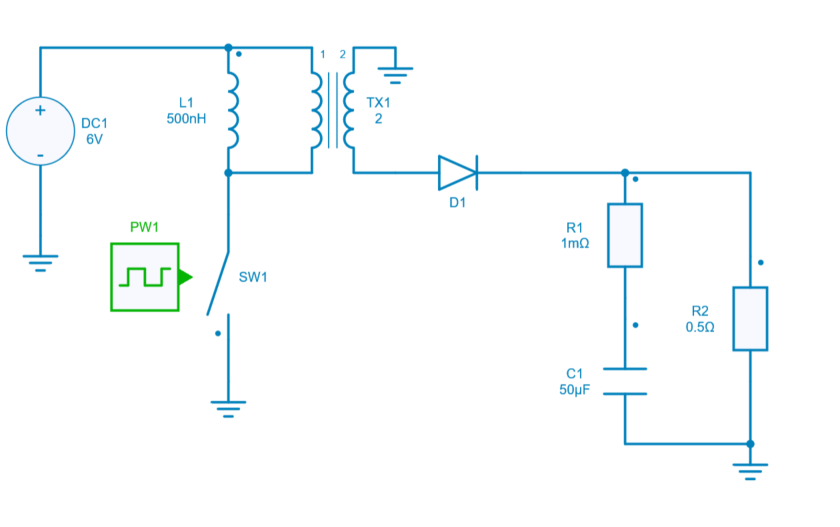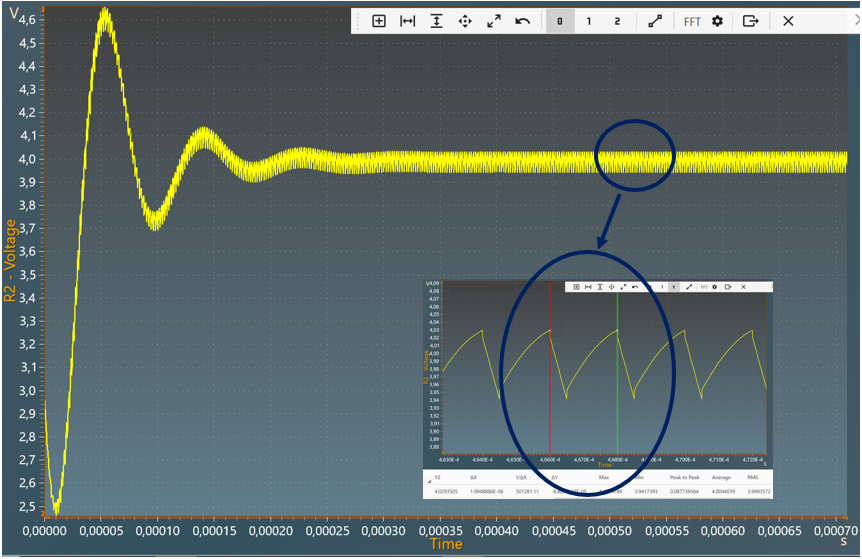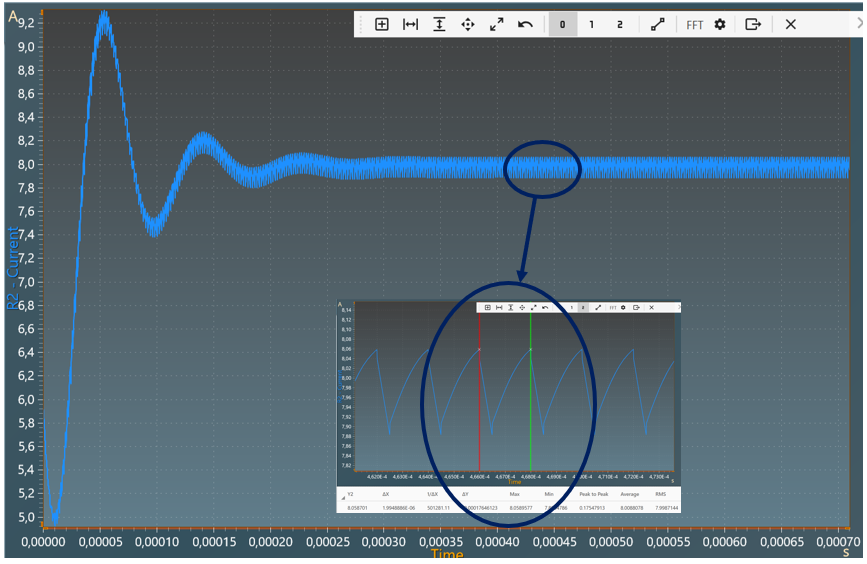Resources

# DC-DC Flyback converter

This example shows a DC-DC Flyback Converter with:

• an input voltage of 6 V,
• an output voltage of 4 V,
• a power of 31.94 W transferred to the output resistor.

The Flyback converter is in reality a power supply based on the principle of an inductive storage chopper, it is a static converter with a galvanic isolation between the input and the output. Its basic scheme is similar to a Buck-Boost converter in which the inductor is replaced by a transformer (actually two coupled inductances). The Flyback converter is generally used in power applications (LCD monitor, CRT TV, DVD player, ...).

## Model

In this model, all components are ideal.In the on-state, the switch is closed, the primary of the transformer is directly connected to the input voltage source. This results in an increase of the magnetic flux in the transformer. The voltage at the secondary boundaries is negative, blocking the diode. It is the output capacitor that provides the energy required by the load.

In the off-state, the switch is opened. The energy stored in the transformer is transferred to the load.

## Switch control

The switch model can be directly connected with a control signal. For this control, we use a PWM block with an amplitude of 1, a duty cycle of 0.25 and a frequency of 500KHz.

Here are the values of the other components :

• inductor L1 : 500nH and initial current = 0A,
• capacitor C1: 50uF and initial voltage = 3V,
• resistor R1: 1 m $$\Omega$$,
• resistor R2: 0.5 $$\Omega$$,
• diode D1: Rd = 0 $$\Omega$$ and Forward voltage = 0V,
• transformer TX1: ratio $$\frac{V2}{V1} = 2$$.

## Simulation

The results below show the output voltage across R2 with a duty cycle= 0.25. A zoom shows a measure of the average value of this voltage : 4 V.The results below show the output current through R2 with a duty cycle= 0.25. A zoom shows a measure of the average value of this current : 8 A.The results follow the equation: $$V_{out} =\frac{N2}{N1} \times V_{in} \times \frac{D}{1-D}$$

with:

• D: Duty cycle
• $$V_{in}$$: Input voltage
• $$V_{out}$$: Output voltage
• N2: Number of turns at secondary
• N1: Number of turns at primary